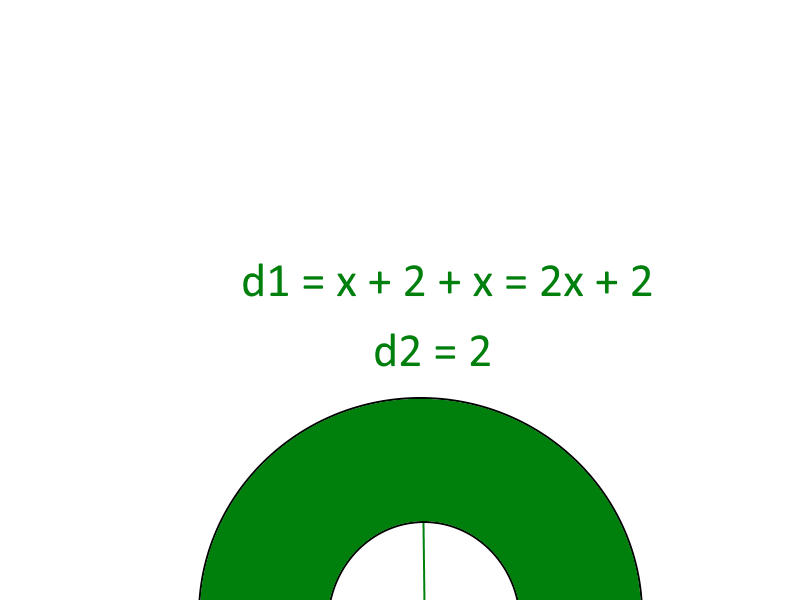My Math ForumCircumference of smaller half circle removed inside of half circle?
 User Name Remember Me? Password

 Algebra Pre-Algebra and Basic Algebra Math Forum

 September 10th, 2018, 09:56 AM #1 Member   Joined: Sep 2014 From: Sweden Posts: 94 Thanks: 0Circumference of smaller half circle removed inside of half circle? So I was trying to find the circumference of a half circle inside of a half circle. By that I mean that I have an empty half circle inside of the larger half circle. So I will need to subtract the smaller half circle at the end.Circumference of half circle: $\displaystyle (\pi * d) / 2$ So assuming what I know is that $\displaystyle d1 = x + 2 + x = 2x + 2$ and that one part in the middle is missing so I only need to add $\displaystyle 2x$. So the equation for O1 (circumference 1) will be: $\displaystyle ((\pi * (2x + 2)) / 2) + 2x$ The diameter of the inner half circle is 2 so therefore: $\displaystyle O2 = ((\pi * 2) / 2) + 2$ $\displaystyle O2 = \pi + 2$ Then I need to subtract O1 with O2 to get the correct circumference. Let's call this O. $\displaystyle O = O1 - O2$ $\displaystyle O = \pi * (x + 1) + 2x - (\pi + 2)$ $\displaystyle O = \pi * (x + 1) + 2x - \pi - 2$ $\displaystyle O = \pi * x + \pi + 2x - \pi - 2$ $\displaystyle O = x(\pi + 2) - 2$ So this is what I get and I don't see where my mistake is. The book's answer is: $\displaystyle (\pi +2)x + 2 * \pi$ Last edited by skipjack; September 10th, 2018 at 03:06 PM.September 10th, 2018, 12:57 PM #2 Math Team   Joined: Oct 2011 From: Ottawa Ontario, Canada Posts: 14,597 Thanks: 1038 What's that link got to do with the problem?September 10th, 2018, 03:30 PM #3 Global Moderator   Joined: Dec 2006 Posts: 20,942 Thanks: 2210 What does $x$ refer to? There's no reason to subtract $O2$ from $O1$. Just add $2x$ to the sum of the lengths of the curved lines: $\pi(x + 1) + \pi + 2x = (\pi + 2)x + 2\pi$.September 14th, 2018, 08:59 AM #4 Member   Joined: Sep 2014 From: Sweden Posts: 94 Thanks: 0 So $\displaystyle \pi$ is the length of the inner half circle's curved line? And then we add the bottom lines of the outer half circle $\displaystyle x + x = 2x$? So what we are doing is taking the curved lines of the outer and inner circle and adding them. Then we add the bottom lines to get the whole circumference. $\displaystyle x$ refers to the bottom lines at the bottom to the left and right. Forgot to specify that. Last edited by DecoratorFawn82; September 14th, 2018 at 09:03 AM.Tags circle, circumference, half, inside, removed, smallerThread ToolsShow Printable VersionEmail this Page Display ModesLinear ModeSwitch to Hybrid ModeSwitch to Threaded ModeSimilar Threads Thread Thread Starter Forum Replies Last Post SlipperyEel Trigonometry 2 June 26th, 2015 03:44 AM fil1977 Calculus 4 April 18th, 2015 06:24 AM Chikis Elementary Math 7 January 22nd, 2014 04:46 PM sir anrava Algebra 1 January 19th, 2010 11:18 AM jimmyfo Algebra 8 May 15th, 2009 09:40 AM

 Contact - Home - Forums - Cryptocurrency Forum - Top

Copyright © 2019 My Math Forum. All rights reserved.